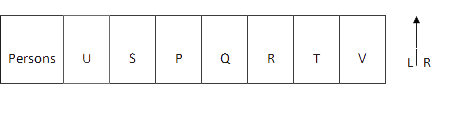# NIIT-Technologies Reasoning Question

DIRECTIONS for questions 1-4: Read the information given below and answer the question that follows.
568   378   654   496   476
1. What will be the resultant if third digit of the highest number is subtracted from the second digit of the smallest number?
1. 3
2. 5
3. 4
4. 2
5. 1
568   378   654   496   476
According to question, highest number of the series is 654. Smallest number of the series is 378. Third digit of the highest number which is 4, is subtracted from the second digit of the smallest number which is 7 then result 7-4=3.
2. If in all the numbers the position of first digit is interchanged with the third digit within the number, how many numbers thus formed will be divisible by 5?
1. Two
2. One
3. Three
4. More than three
5. None
If in all the numbers the position of first digit is interchanged with the third digit within the number, the series is 865 873  456 694  674
Only 865 is the number which is divisible by 5.
1. If all the digits in each number are arranged in descending order within the number and the numbers thus formed are arranged in descending order from left to right, which of the following will be the third number from the left end?
1. 568
2.  476
3. 378
4. 496
5. 654
If all the digits in each number are arranged in descending order within the number and the numbers thus formed are arranged in descending order from left to right, then series is 964 973 865 764 654
865 is the third number from left. Therefore, 568 is answer.
2. If 1 is subtracted from every even digit in each of the numbers, how many numbers thus formed will have a digit appear twice?
1. None
2. More than three
3. One
4. Two
5. Three
If 1 is subtracted from every even digit in each of the numbers, Series is :
557 377 553 395 375
Thus, there are 3 numbers which have a digit appearing twice (i.e., 557, 377 and 553).
3. If '2' is added to the first digit of each number and '2' is subtracted from the middle digit and third digit of each number, the sum of digits of how many numbers thus formed will be equal to 17?
1. Four
2. Two
3. None
4. One
5. Three
If '2' is added to the first digit of each number and '2' is subtracted from the middle digit and third digit of each number, then series is,
746  556  832  674  654
7+4+6=17 & 6+7+4=17. There are two numbers whose sum of digits is 17.
4. There are thirty students in a class. Prakash ranks third among the boys. Priya ranks fifth among the girls. Prakash is one rank below Priya in the class. No two students hold the same rank. What is Priya’s rank in the class?
1. X
2.  P
3. Z
4. I
5. None of these
Meaningful word can be made from P, I & T are PIT and TIP. Since, more than one such word can be formed thus correct answer is Z.
DIRECTIONS for questions 7-10:Read the information given below and answer the question that follows.
Seven people- P, Q, R, S, T, U and V are sitting in a straight line facing north with equal distances between each other but not necessarily in the same order.
Q sits exactly in the middle of the line. R is an immediate neighbor of O. Only three people sit between U and R. S is an immediate neighbor of U. T sits fourth to the right of S. P sits at one of the positions to the left of T.
5. As per the given arrangement, four of the following five are alike in a certain way and hence form a group. Which of the following does not belong to the group?
1. VT
2.  RT
3. SU
4. QP
5. PTAs per the given arrangement, PT does not belong to the group, because both persons in all other options are immediate neighbor of each other.
6. Who sits exactly between R and V?
1. P
2.  S
3. Q
4. T
5. Other than those given as options
T sits exactly between R and V.
7. What is the position of S with respect to P?
1. Second to the left
2. Second to the right
3.  Immediate right
4. Immediate left
5. Third to the right